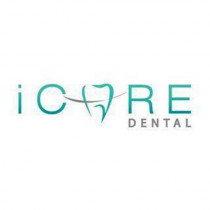iCare 牙科诊所（Taman Connaught）马来西亚，吉隆坡，蕉赖 Cheras

100%推荐，51条评论
• 牙科

＋展开全部

简介

iCare 牙科诊所提供舒适愉快的治疗体验和全方位优质的疗程，包括普通牙科、预防程序、牙周治疗、 口腔外科手术、 牙髓根管治疗、 冠桥修复、牙套和牙科植入物。

1. 高质量的现代牙科尤其是牙科植入物和牙科美学。
2. 最好的牙科经验。
3. 感染控制和安全标准。
4. 采用最新的牙科技术和治疗方式。
＋展开全部

地点 & 门诊时间 目前营业 目前休息

108 Mezzanine Floor
Jalan Cerdas, Taman Connaught,
56000 Cheras10:00 - 18:00

10:00 - 18:00

10:00 - 16:00

10:00 - 18:00

10:00 - 18:00

10:00 - 18:00

牙齿矫正（绑牙套）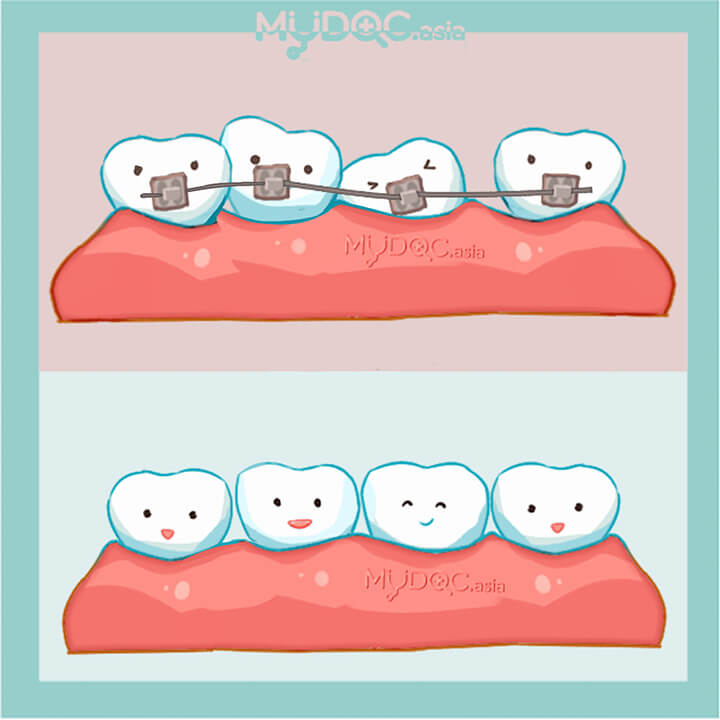• 弯曲的牙齿
• 牙齿不均匀
• 深覆牙合
• 反颌咬合
• 咀嚼问题

传统金属牙套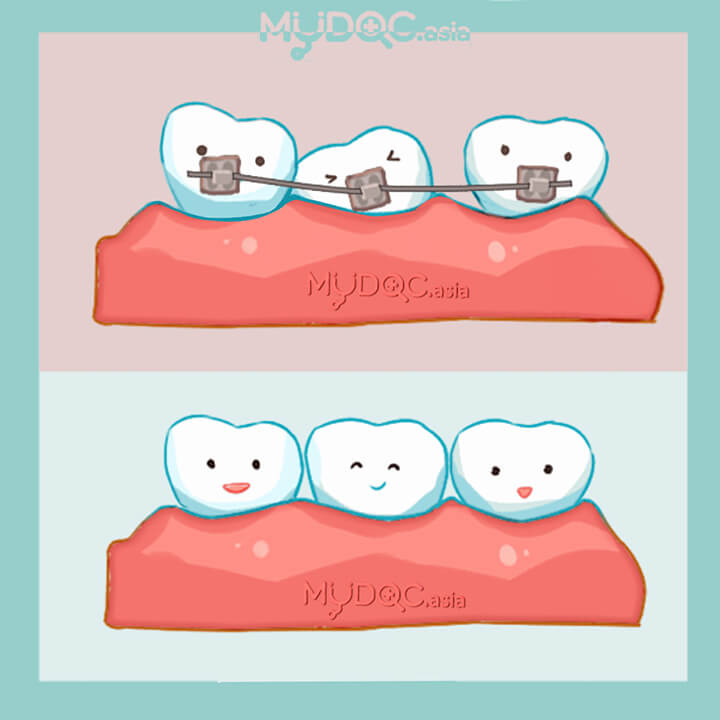• 弯曲的牙齿
• 牙齿不均匀
• 深覆牙合
• 反颌咬合
• 咀嚼问题

陶瓷牙套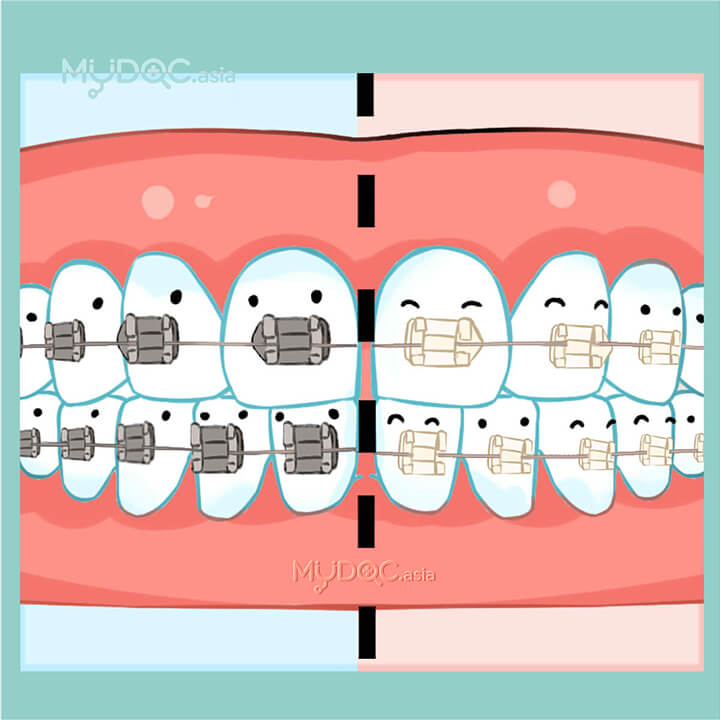• 牙齿不均匀
• 深覆牙合
• 反颌咬合
• 咀嚼问题
• 弯曲的牙齿

透明牙套（隐形牙套）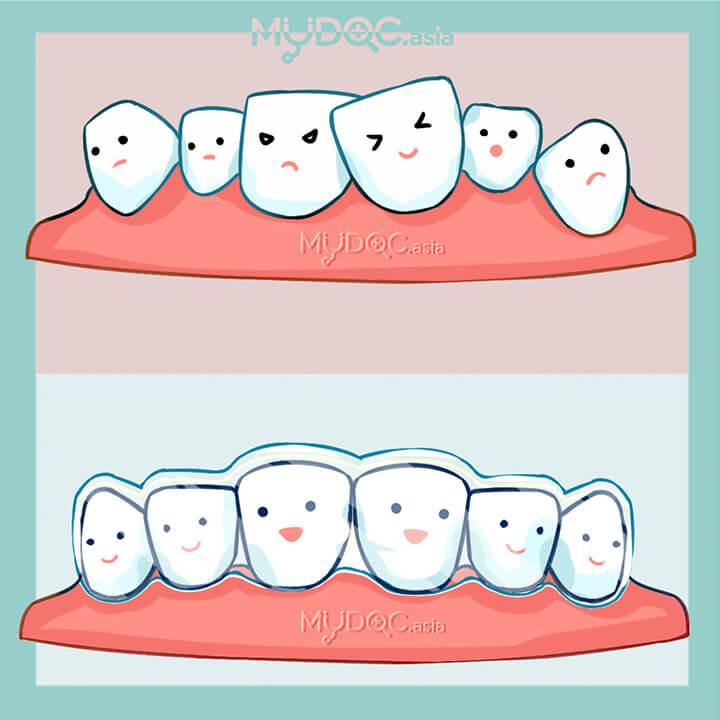• 弯曲的牙齿
• 牙齿不均匀
• 深覆牙合
• 反颌咬合
• 咀嚼问题

隐适美（Invisalign）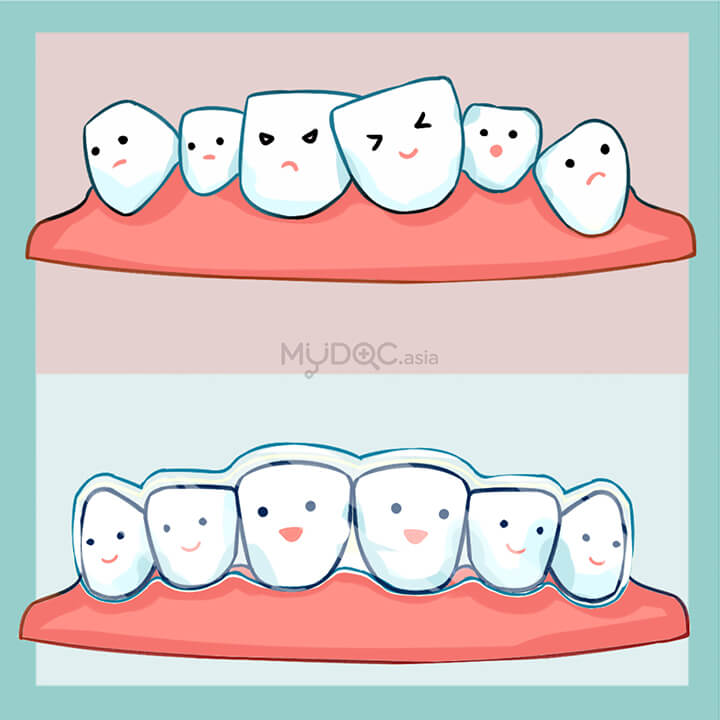• 牙齿不均匀
• 弯曲的牙齿
• 深覆牙合
• 反颌咬合
• 咀嚼问题

舌侧牙套（隐形牙套）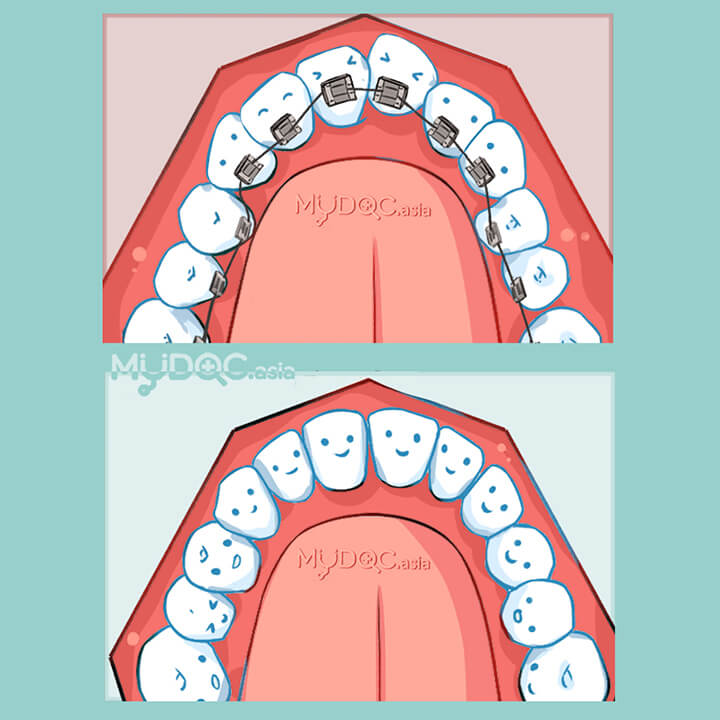• 弯曲的牙齿
• 牙齿不均匀
• 深覆牙合
• 反颌咬合
• 咀嚼问题

自锁式牙套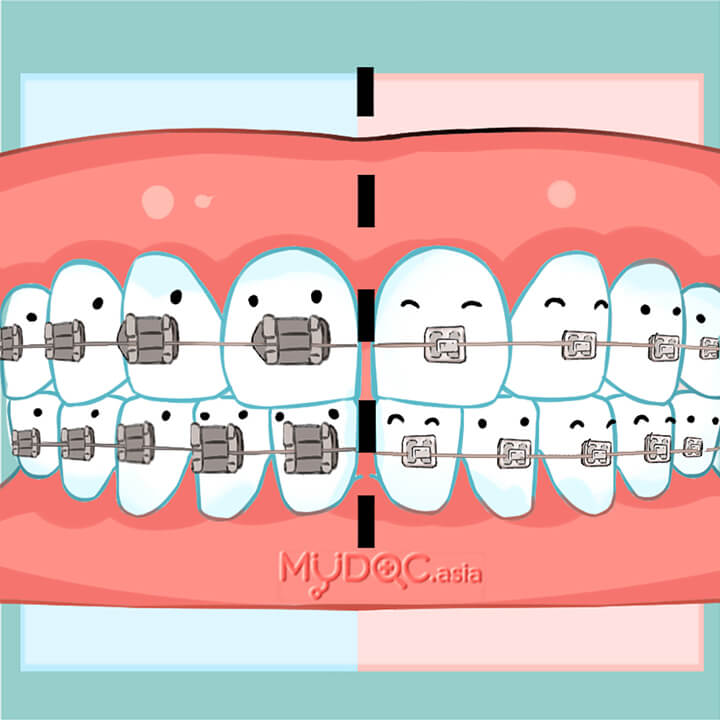• 牙齿不均匀
• 深覆牙合
• 反颌咬合
• 咀嚼问题
• 弯曲的牙齿

Damon自锁牙套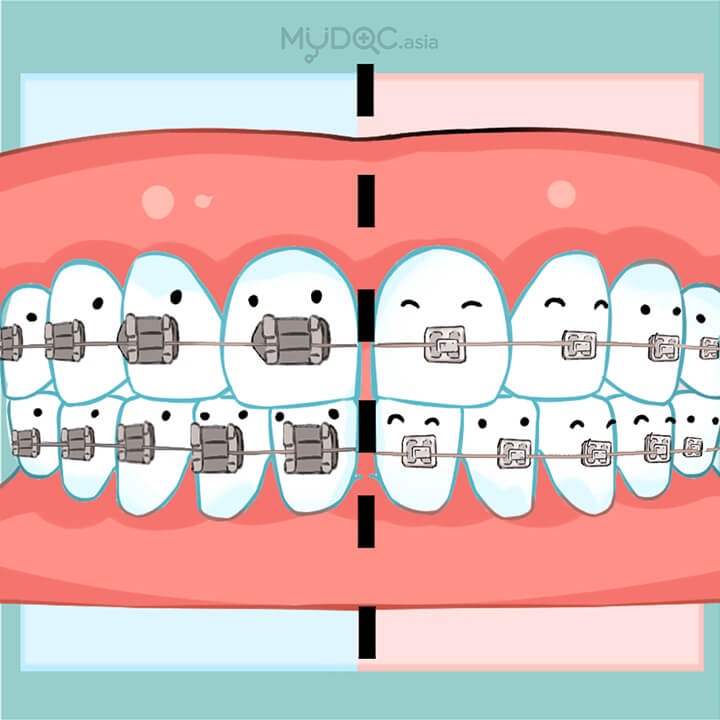• 牙齿不均匀
• 深覆牙合
• 反颌咬合
• 咀嚼问题
• 弯曲的牙齿

加速式牙齿矫正（牙套）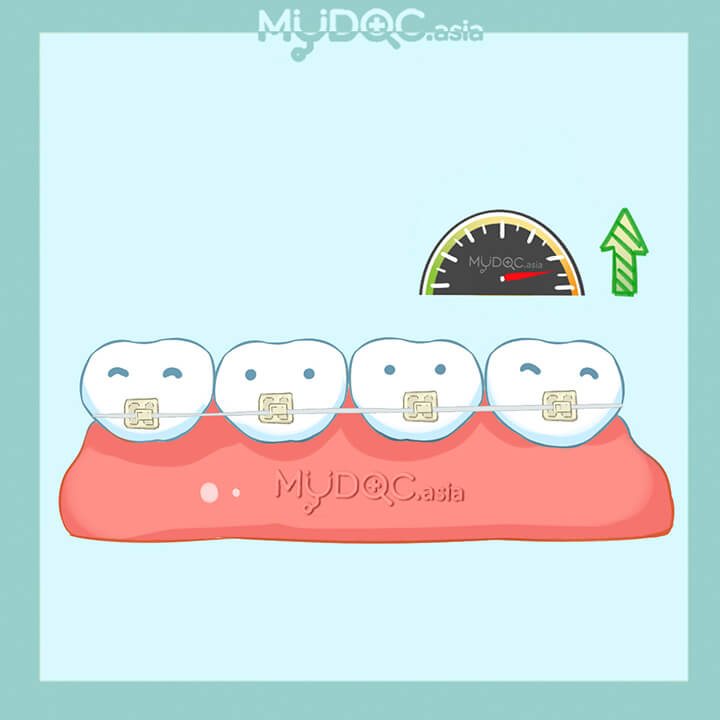• 牙齿不均匀
• 深覆牙合
• 反颌咬合
• 咀嚼问题
• 弯曲的牙齿

Six Month Smiles 快速牙齿矫正牙套• 牙齿不均匀
• 深覆牙合
• 反颌咬合
• 咀嚼问题
• 弯曲的牙齿

护牙套• 磨牙
• 睡眠呼吸暂停
• 颞颌关节症（TMJ）
• 体育相关的牙齿损伤
• 牙齿不均匀
• 深覆牙合
• 反颌咬合
• 咀嚼问题
• 弯曲的牙齿

护齿套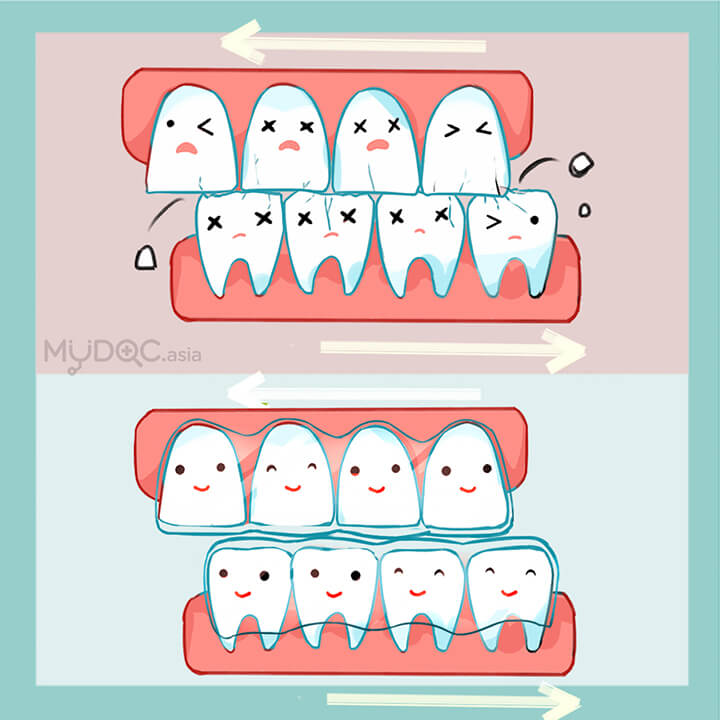• 磨牙

牙齿保持器／固定器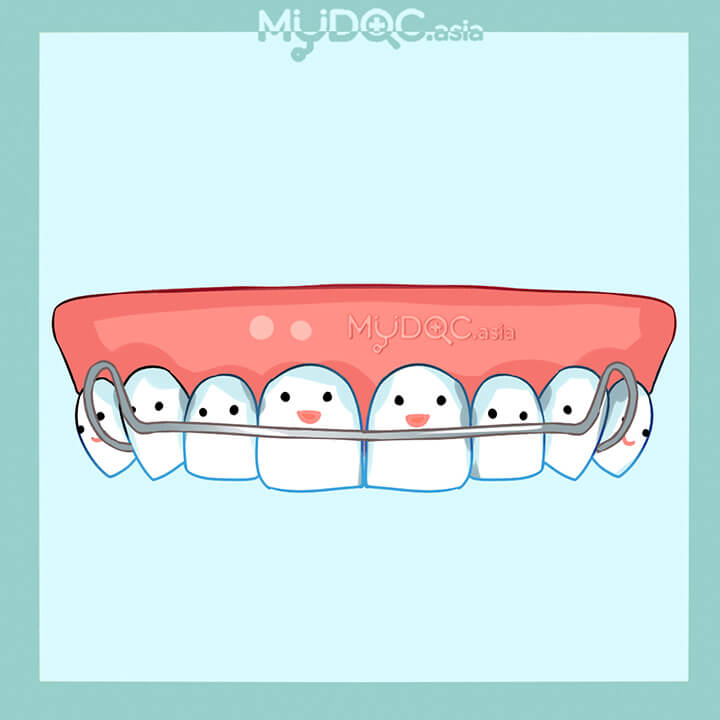• 弯曲的牙齿
• 牙齿不均匀
• 深覆牙合
• 反颌咬合
• 咀嚼问题

固定保持器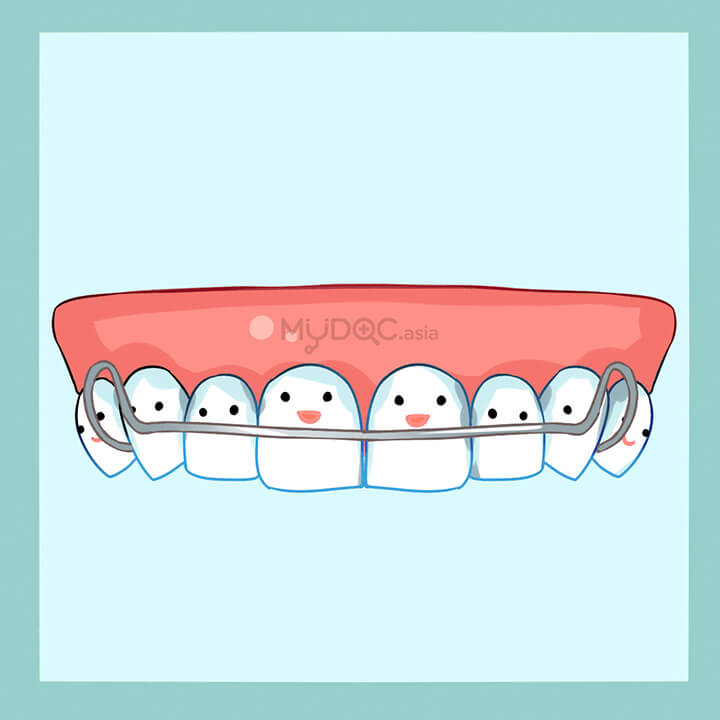• 牙齿不均匀
• 弯曲的牙齿
• 深覆牙合
• 反颌咬合
• 咀嚼问题

透明保持器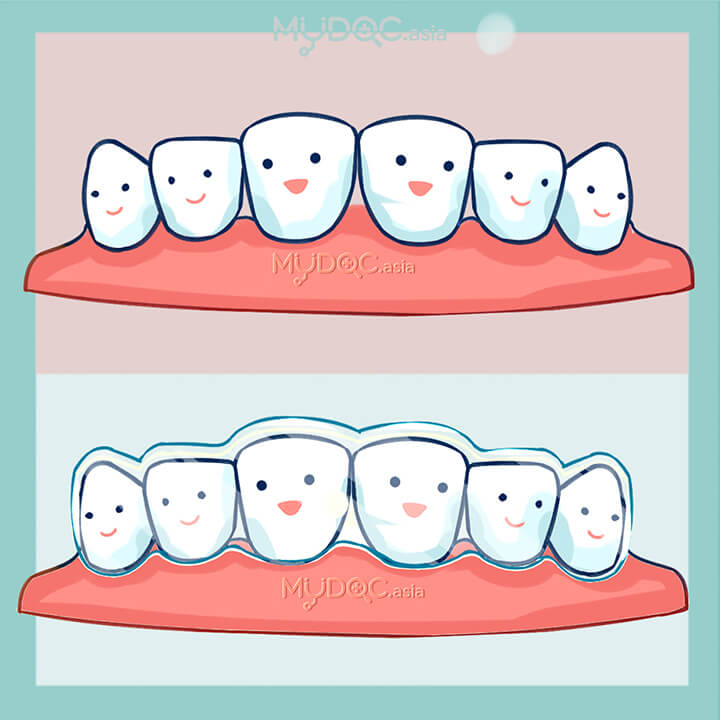• 牙齿不均匀
• 弯曲的牙齿
• 深覆牙合
• 反颌咬合
• 咀嚼问题

哈雷保持器• 牙齿不均匀
• 弯曲的牙齿
• 深覆牙合
• 反颌咬合
• 咀嚼问题

美白牙齿貼面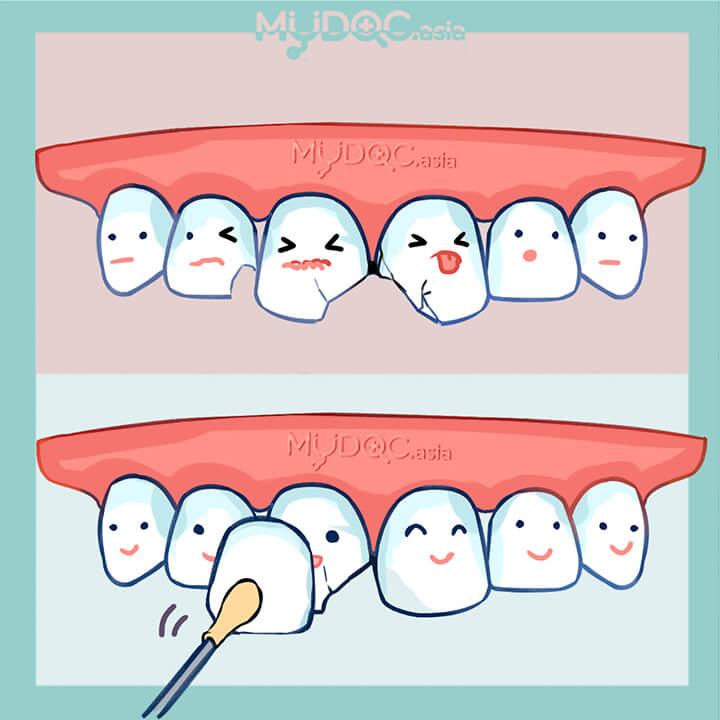• 牙齿侵蚀症
• 蛀牙
• 牙齿损伤
• 牙洞
• 牙齿断裂
• 牙齿破裂

烤瓷牙贴面• 牙齿侵蚀症
• 蛀牙
• 牙齿损伤
• 牙洞
• 牙齿断裂
• 牙齿破裂

二氧化锆牙贴面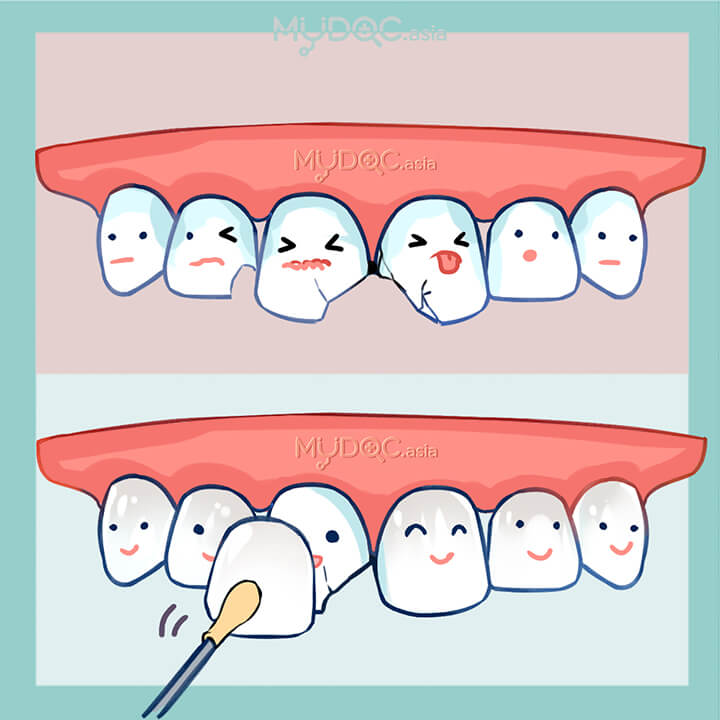• 牙齿损伤
• 牙齿断裂
• 牙洞
• 牙齿破裂

Lumineers 舒芙瓷美白牙贴面• 牙齿损伤
• 牙洞
• 牙齿断裂
• 牙洞

牙冠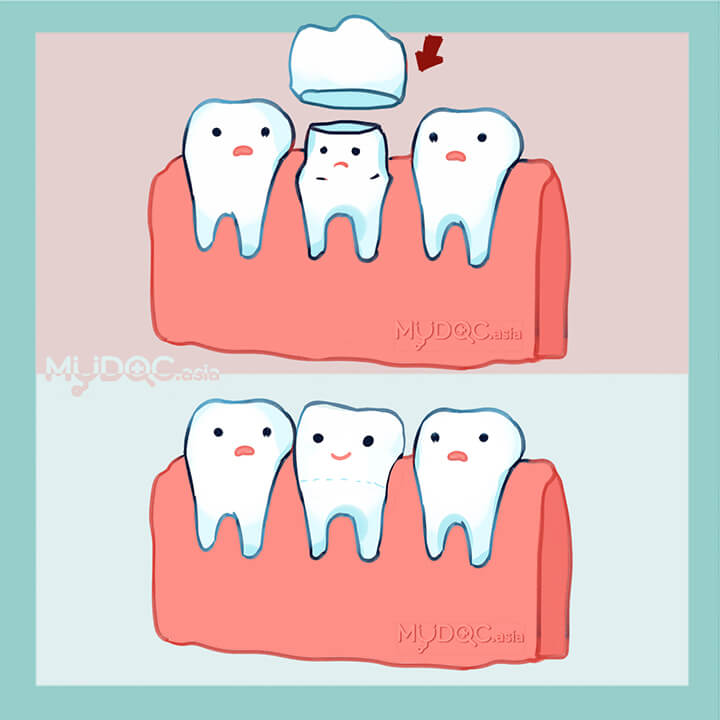• 蛀牙
• 牙齿侵蚀症
• 牙科疾病
• 牙齿损伤
• 牙洞
• 牙齿断裂
• 牙齿破裂

瓷牙冠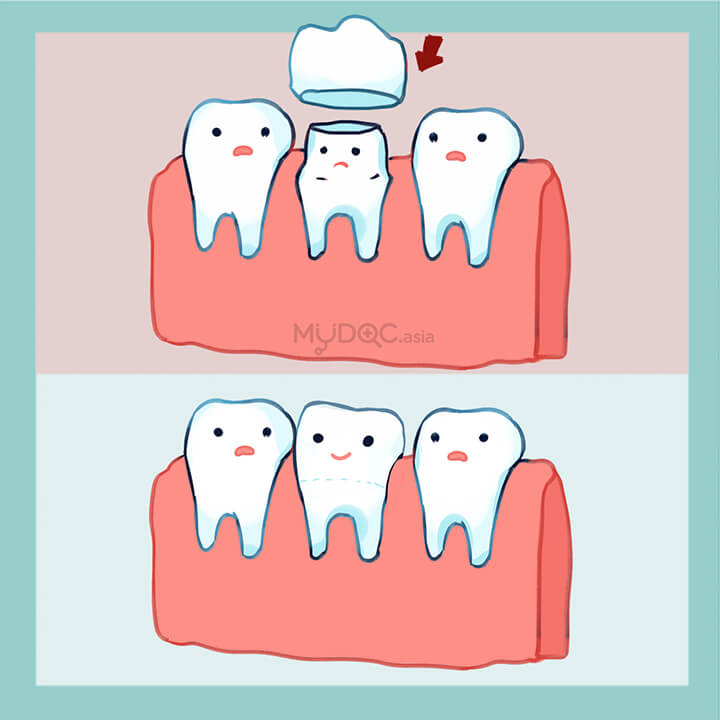• 蛀牙
• 牙齿侵蚀症
• 牙科疾病
• 牙齿损伤
• 牙洞
• 牙齿断裂
• 牙齿破裂

烤瓷熔附金属（PFM）牙冠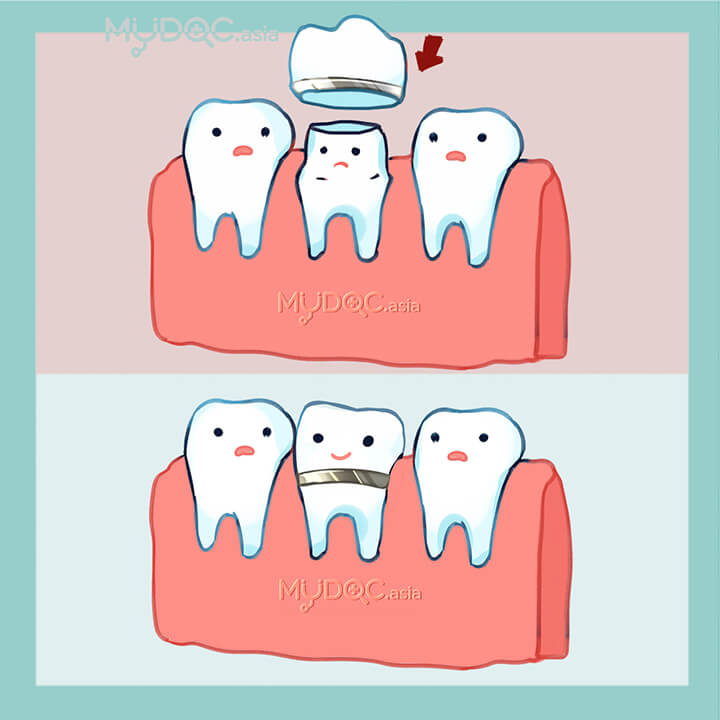• 牙科疾病
• 蛀牙
• 牙齿侵蚀症
• 牙齿损伤
• 牙洞
• 牙齿破裂
• 牙齿断裂

二氧化锆牙冠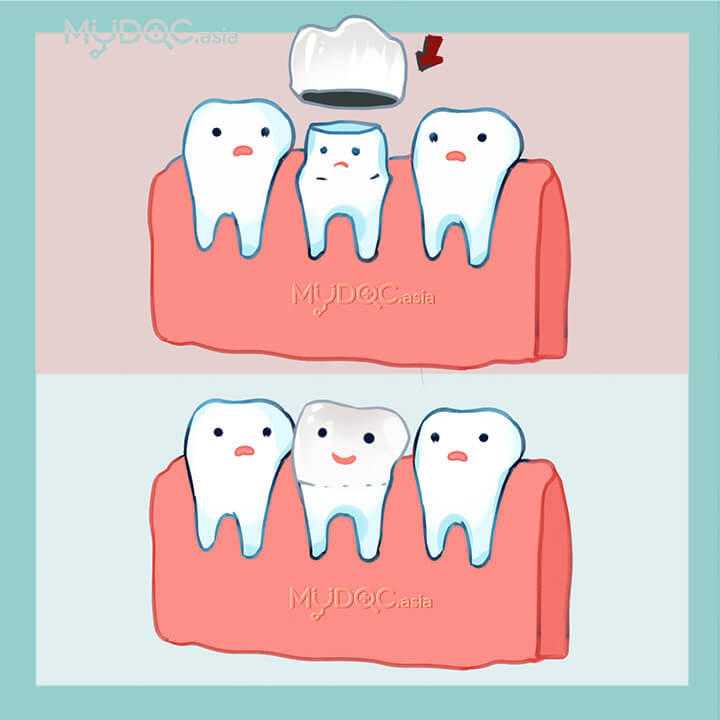• 牙齿侵蚀症
• 牙科疾病
• 蛀牙
• 牙洞
• 牙齿断裂
• 牙齿破裂
• 牙齿损伤

根尖切除• 牙科疾病
• 牙髓病
• 牙根炎
• 牙根感染

假牙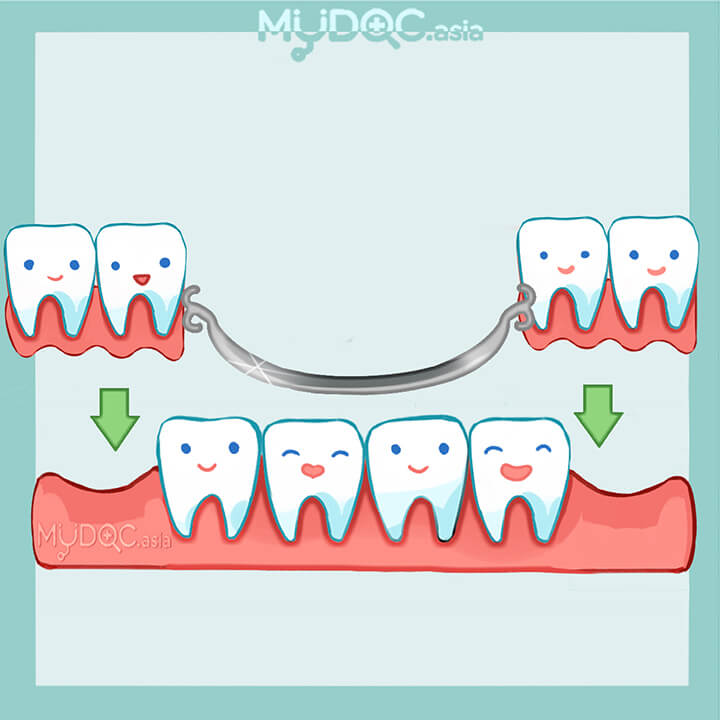• 牙齿碎裂
• 缺牙
• 牙齿脱落
• 更换牙齿

亚克力假牙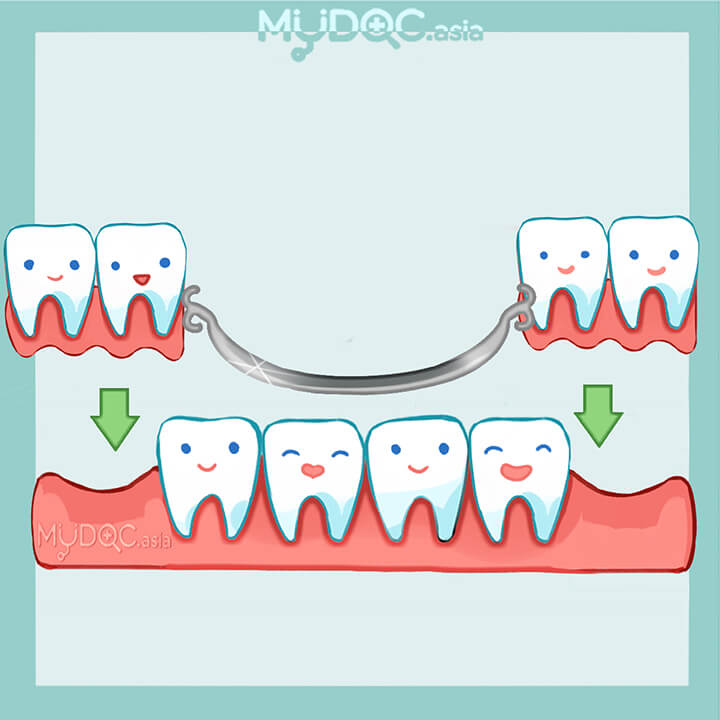• 牙齿碎裂
• 缺牙
• 牙齿脱落
• 更换牙齿

钴铬合金义齿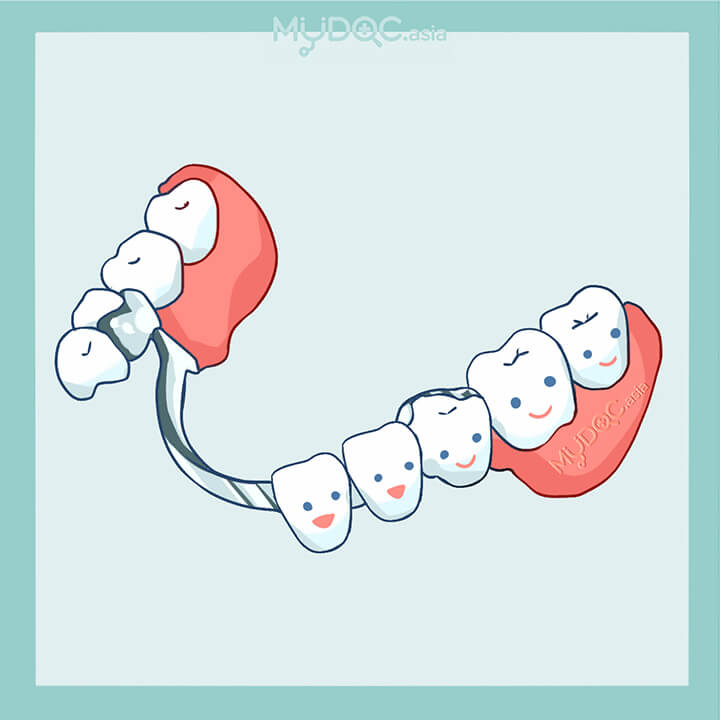• 牙齿碎裂
• 缺牙
• 牙齿脱落
• 更换牙齿

全口假牙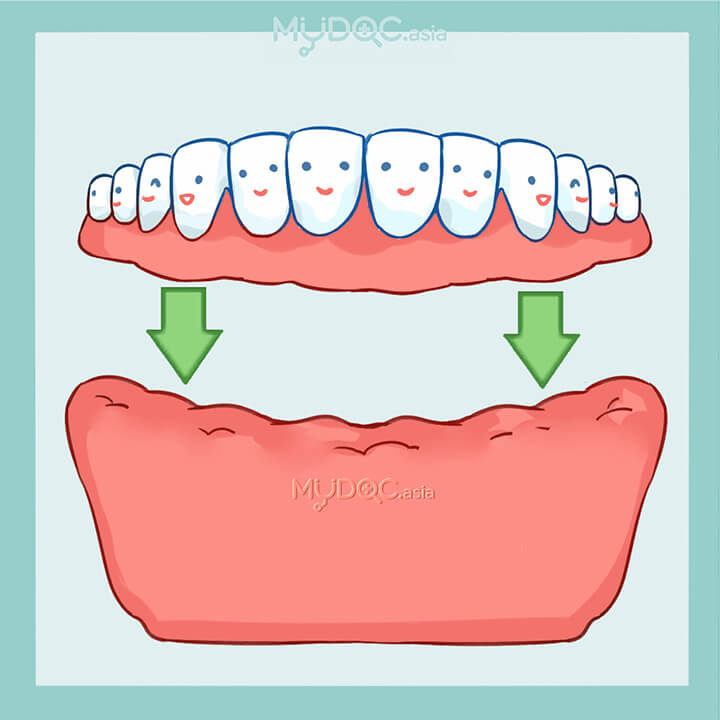• 牙齿碎裂
• 缺牙
• 牙齿脱落
• 更换牙齿

假牙修复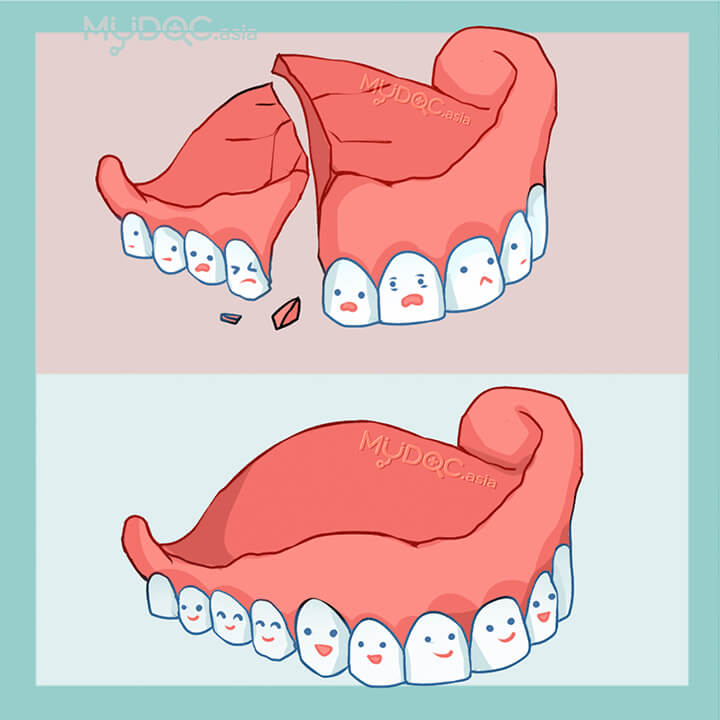• 假牙破裂
• 牙齿脱落
• 缺牙
• 更换牙齿

种植牙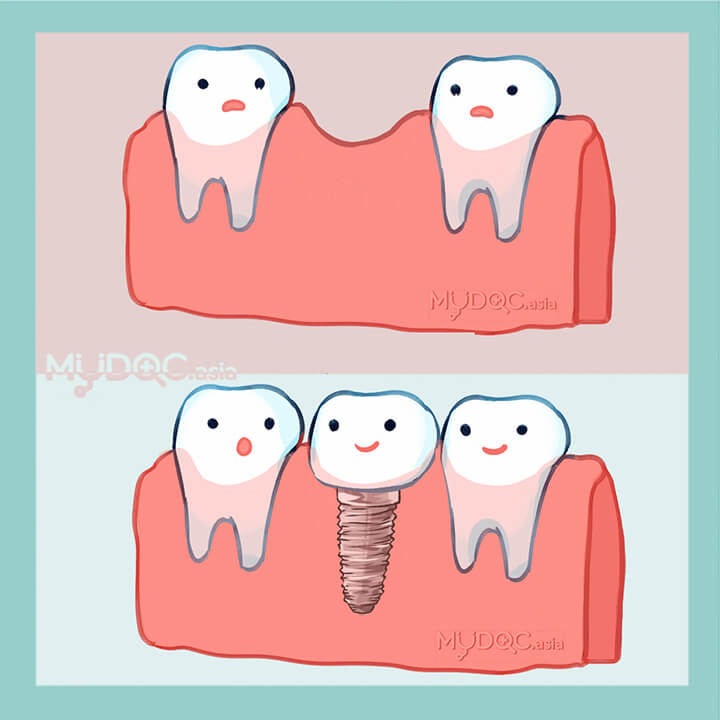• 牙齿碎裂
• 缺牙
• 牙齿脱落
• 更换牙齿

钛合金种植牙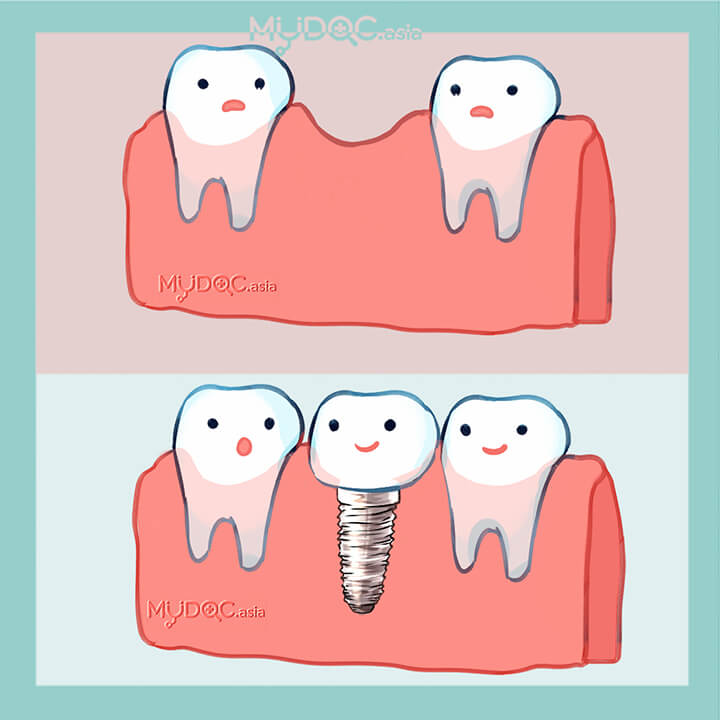• 牙齿脱落
• 缺牙
• 更换牙齿

迷你植牙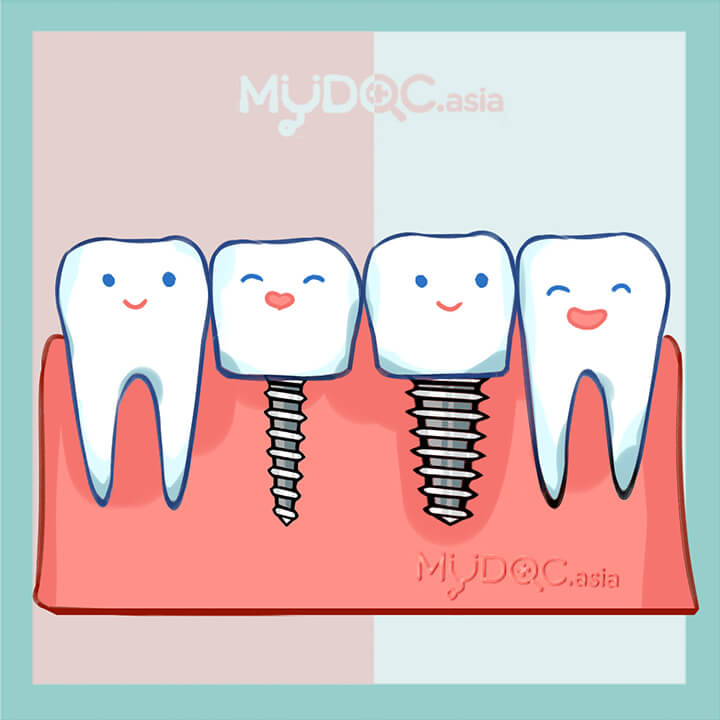• 牙齿脱落
• 缺牙
• 更换牙齿

All on4 全口植牙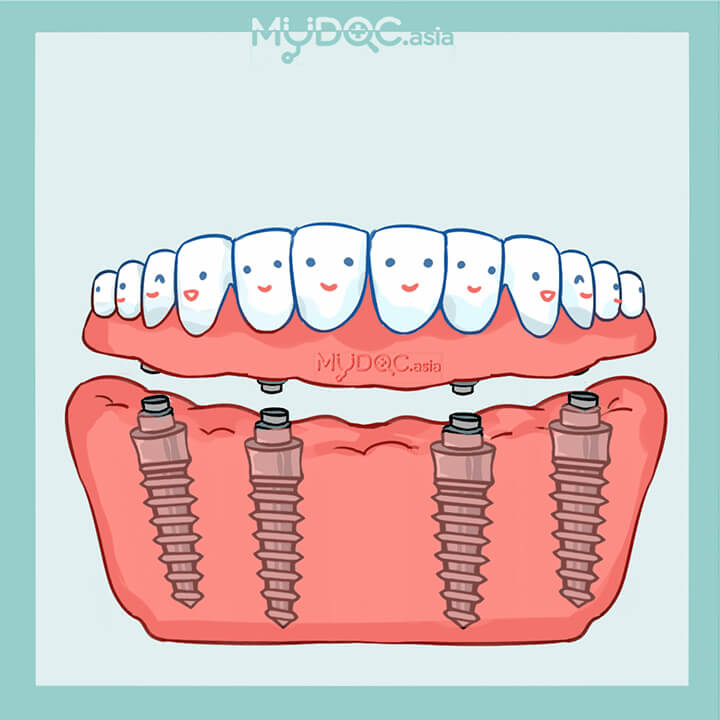• 牙齿脱落
• 缺牙
• 更换牙齿

All on 6 全口植牙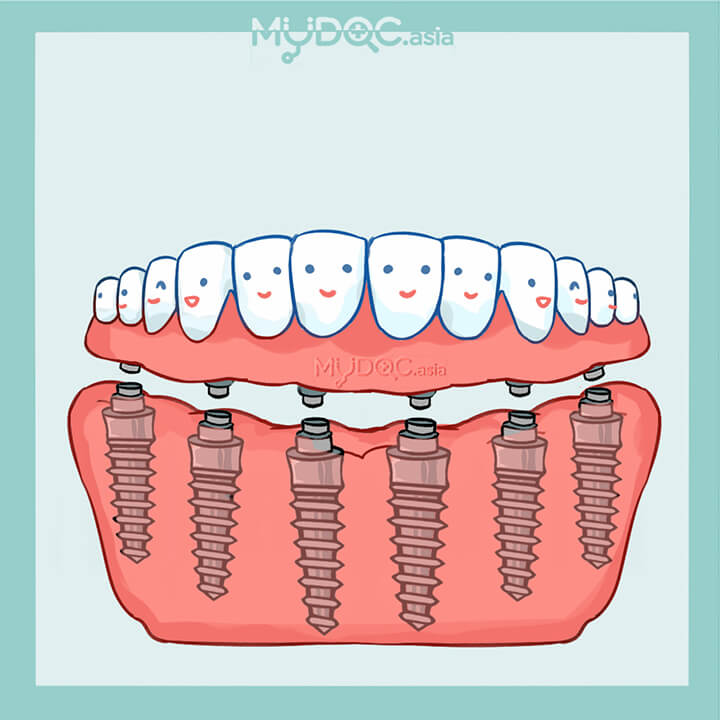• 牙齿脱落
• 缺牙
• 更换牙齿

补牙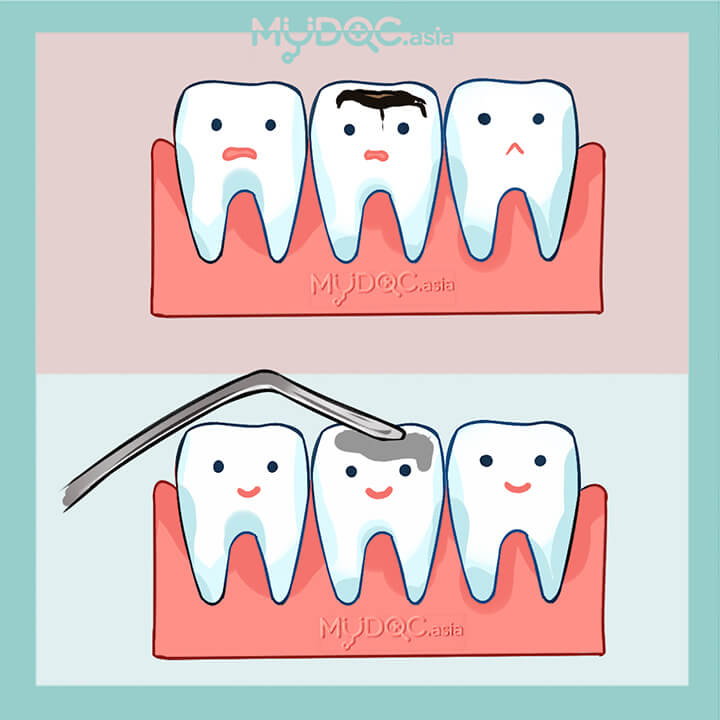• 蛀牙
• 牙科疾病
• 牙齿损伤
• 牙洞
• 牙齿断裂
• 牙齿破裂

银汞合金补牙• 蛀牙
• 牙科疾病
• 牙齿损伤
• 牙洞
• 牙齿断裂
• 牙齿破裂

复合树脂补牙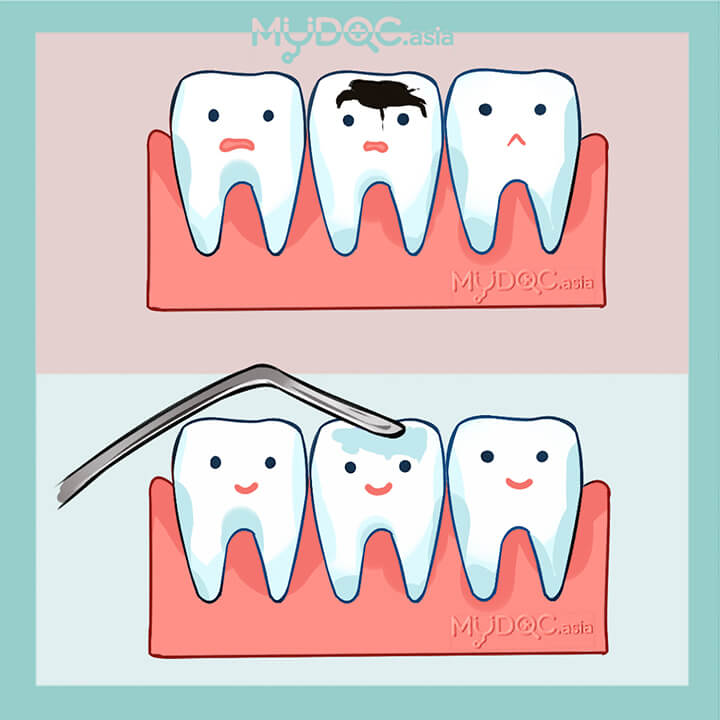• 蛀牙
• 牙科疾病
• 牙齿损伤
• 牙洞
• 牙齿断裂
• 牙齿破裂

牙桥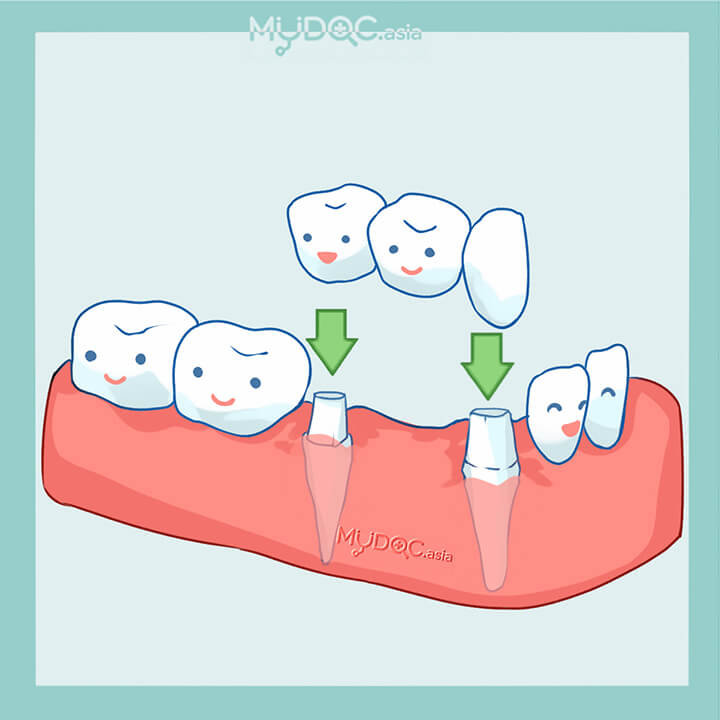• 缺牙
• 牙齿脱落
• 更换牙齿

马利兰牙桥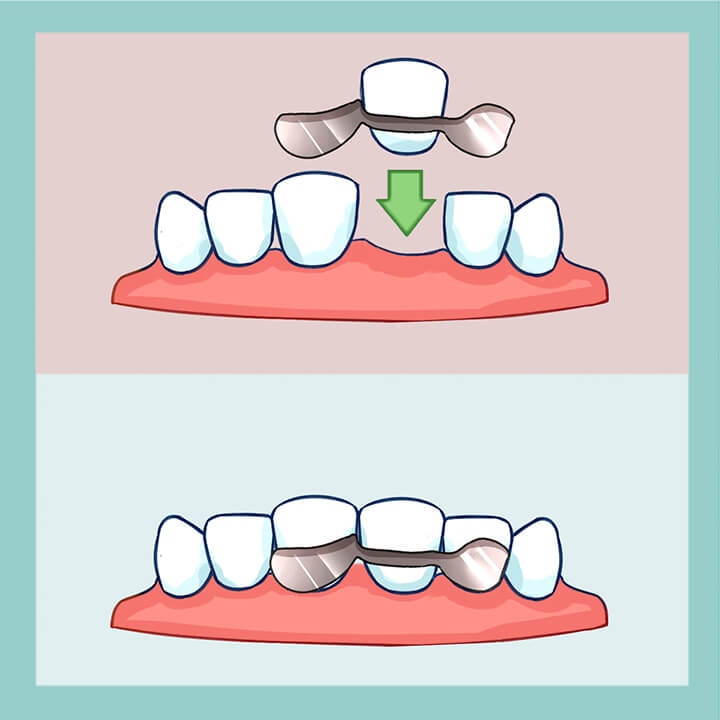• 缺牙
• 牙齿脱落
• 更换牙齿

悬臂式牙桥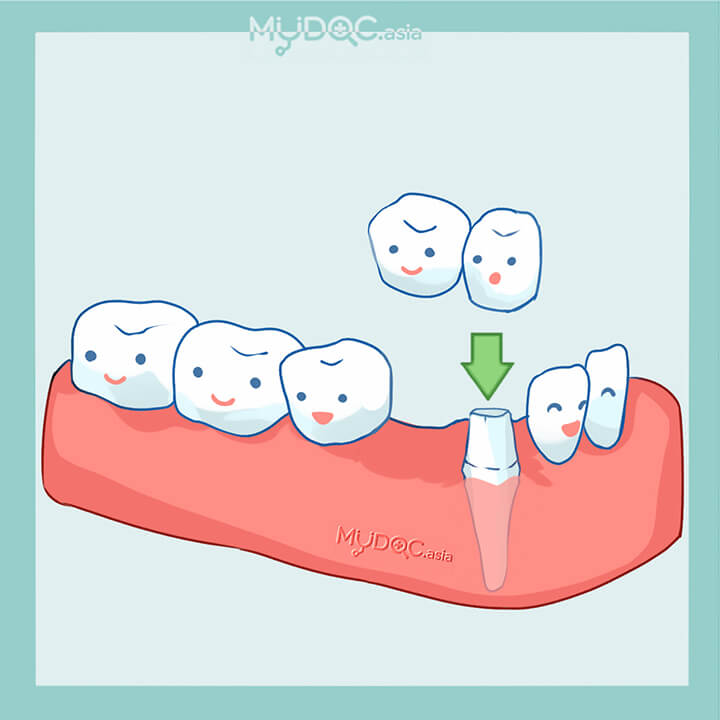• 缺牙
• 牙齿脱落
• 更换牙齿

传统牙桥• 缺牙
• 牙齿脱落
• 更换牙齿

陶瓷填充式牙齿修复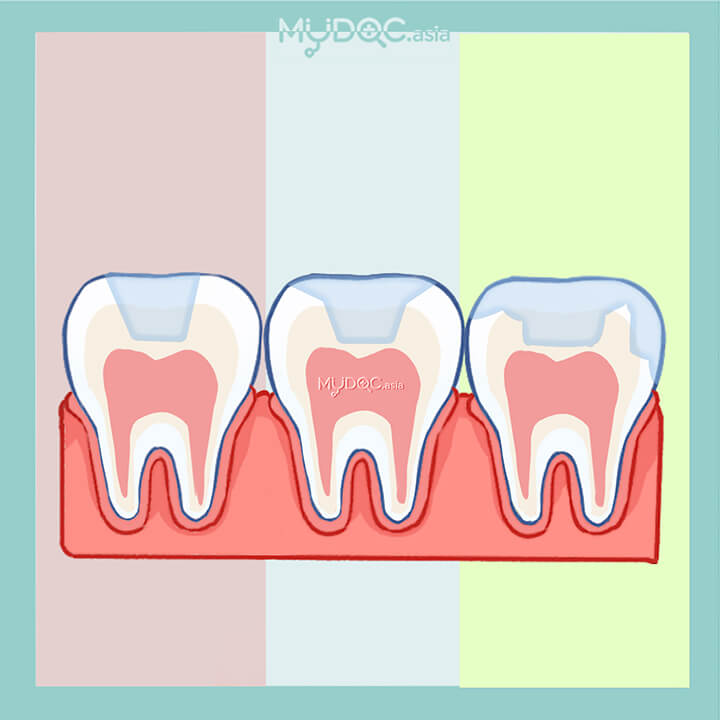• 蛀牙
• 牙科疾病
• 牙齿损伤
• 牙洞
• 牙齿断裂
• 牙齿破裂

牙齿美白疗程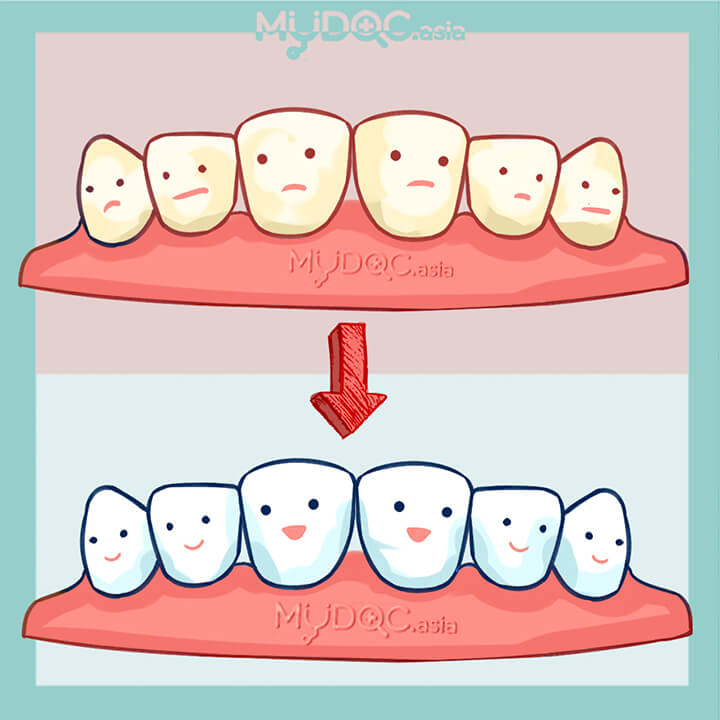• 牙齿染色
• 牙齿变色
• 牙齿发黄

激光美白牙齿• 牙齿染色
• 牙齿变色
• 牙齿发黄

牙齿美白仪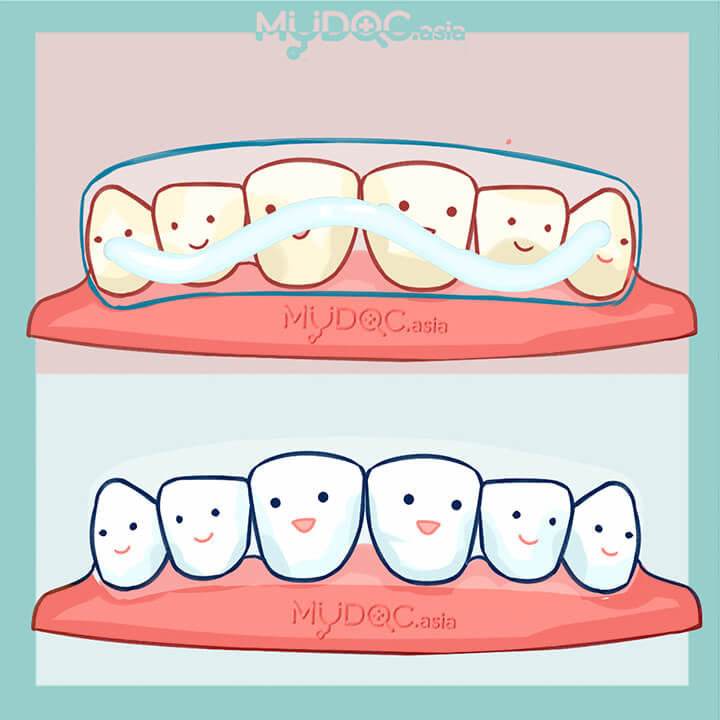• 牙齿染色
• 牙齿变色
• 牙齿发黄

牙桥• 缺牙
• 牙齿脱落
• 更换牙齿

马利兰牙桥• 缺牙
• 牙齿脱落
• 更换牙齿

悬臂式牙桥• 缺牙
• 牙齿脱落
• 更换牙齿

传统牙桥• 缺牙
• 牙齿脱落
• 更换牙齿

种植牙• 牙齿碎裂
• 缺牙
• 牙齿脱落
• 更换牙齿

迷你植牙• 牙齿脱落
• 缺牙
• 更换牙齿

钛合金种植牙• 牙齿脱落
• 缺牙
• 更换牙齿

All on4 全口植牙• 牙齿脱落
• 缺牙
• 更换牙齿

All on 6 全口植牙• 牙齿脱落
• 缺牙
• 更换牙齿

美白牙齿貼面• 牙齿侵蚀症
• 蛀牙
• 牙齿损伤
• 牙洞
• 牙齿断裂
• 牙齿破裂

烤瓷牙贴面• 牙齿侵蚀症
• 蛀牙
• 牙齿损伤
• 牙洞
• 牙齿断裂
• 牙齿破裂

二氧化锆牙贴面• 牙齿损伤
• 牙齿断裂
• 牙洞
• 牙齿破裂

Lumineers 舒芙瓷美白牙贴面• 牙齿损伤
• 牙洞
• 牙齿断裂
• 牙洞

牙冠• 蛀牙
• 牙齿侵蚀症
• 牙科疾病
• 牙齿损伤
• 牙洞
• 牙齿断裂
• 牙齿破裂

瓷牙冠• 蛀牙
• 牙齿侵蚀症
• 牙科疾病
• 牙齿损伤
• 牙洞
• 牙齿断裂
• 牙齿破裂

烤瓷熔附金属（PFM）牙冠• 牙科疾病
• 蛀牙
• 牙齿侵蚀症
• 牙齿损伤
• 牙洞
• 牙齿破裂
• 牙齿断裂

二氧化锆牙冠• 牙齿侵蚀症
• 牙科疾病
• 蛀牙
• 牙洞
• 牙齿断裂
• 牙齿破裂
• 牙齿损伤

假牙• 牙齿碎裂
• 缺牙
• 牙齿脱落
• 更换牙齿

亚克力假牙• 牙齿碎裂
• 缺牙
• 牙齿脱落
• 更换牙齿

钴铬合金义齿• 牙齿碎裂
• 缺牙
• 牙齿脱落
• 更换牙齿

全口假牙• 牙齿碎裂
• 缺牙
• 牙齿脱落
• 更换牙齿

补牙• 蛀牙
• 牙科疾病
• 牙齿损伤
• 牙洞
• 牙齿断裂
• 牙齿破裂

银汞合金补牙• 蛀牙
• 牙科疾病
• 牙齿损伤
• 牙洞
• 牙齿断裂
• 牙齿破裂

复合树脂补牙• 蛀牙
• 牙科疾病
• 牙齿损伤
• 牙洞
• 牙齿断裂
• 牙齿破裂

陶瓷填充式牙齿修复• 蛀牙
• 牙科疾病
• 牙齿损伤
• 牙洞
• 牙齿断裂
• 牙齿破裂

儿童牙科密封剂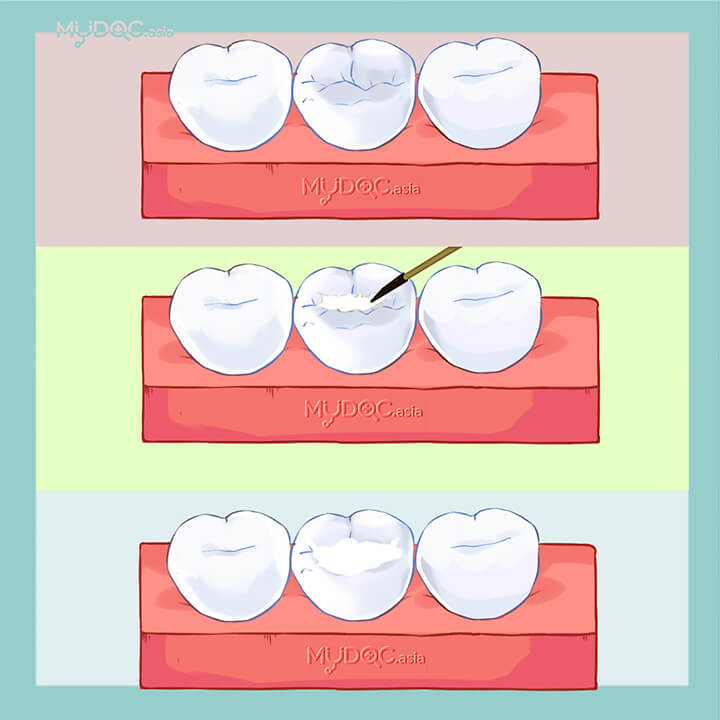• 蛀牙
• 牙科疾病
• 牙齿损伤
• 牙齿感染
• 齿斑块
• 牙结石

儿科补牙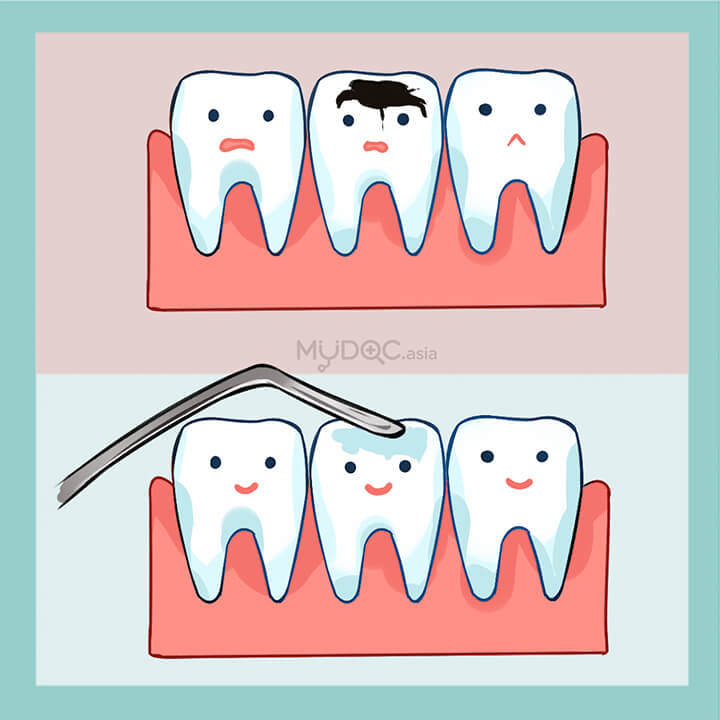• 牙科疾病
• 蛀牙
• 牙齿损伤
• 牙洞
• 牙齿断裂
• 牙齿破裂

• 蛀牙
• 牙科疾病
• 牙齿松动

洗牙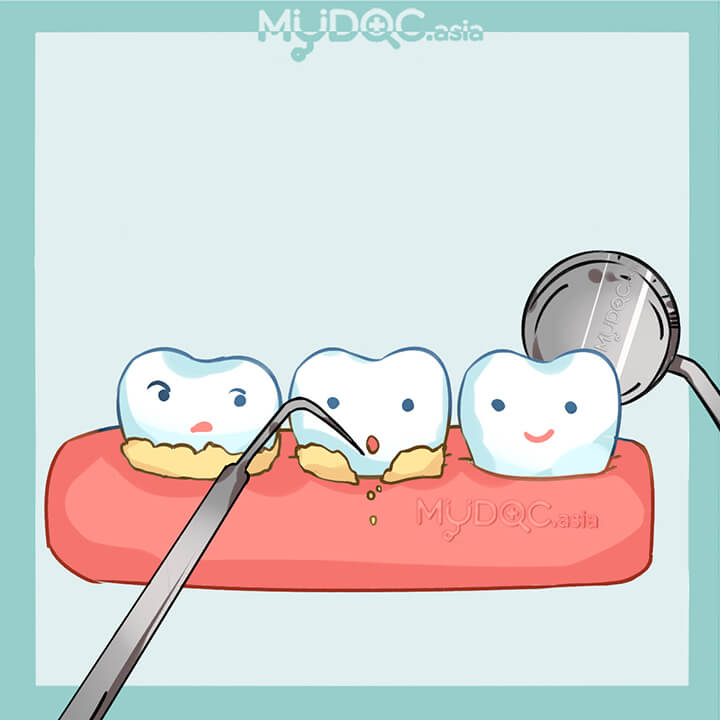• 齿斑块
• 牙齿染色
• 蛀牙
• 牙齿损伤
• 牙齿感染
• 牙结石

牙氟化物治疗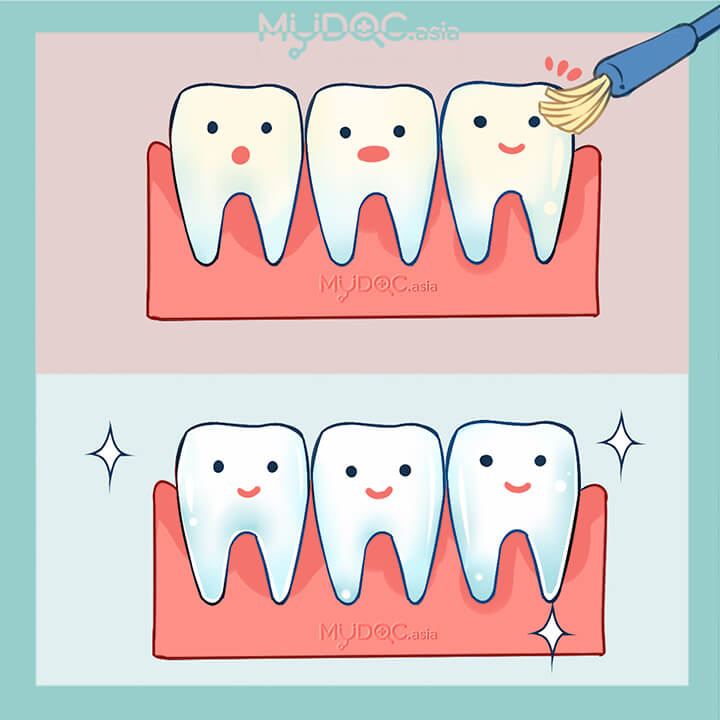• 蛀牙
• 牙科疾病
• 牙齿损伤
• 牙齿感染
• 齿斑块
• 牙结石

牙病预防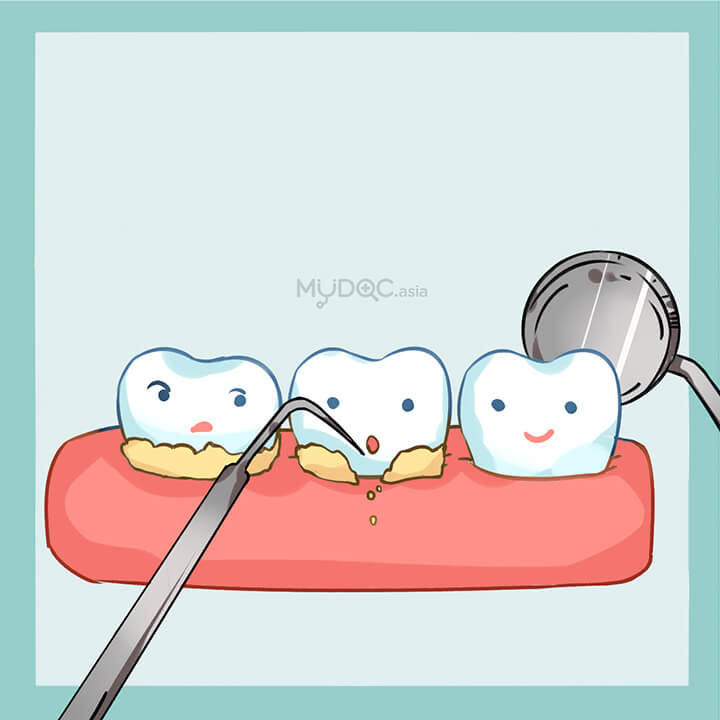• 牙周病
• 牙龈炎
• 牙科疾病
• 蛀牙
• 牙齿损伤
• 牙齿感染
• 齿斑块
• 牙结石

牙科密封剂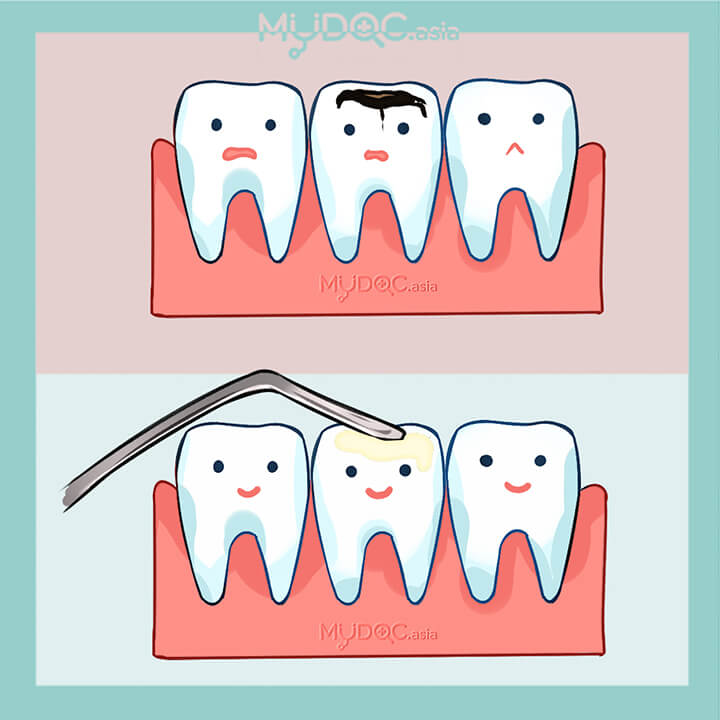• 蛀牙
• 牙科疾病

牙医咨询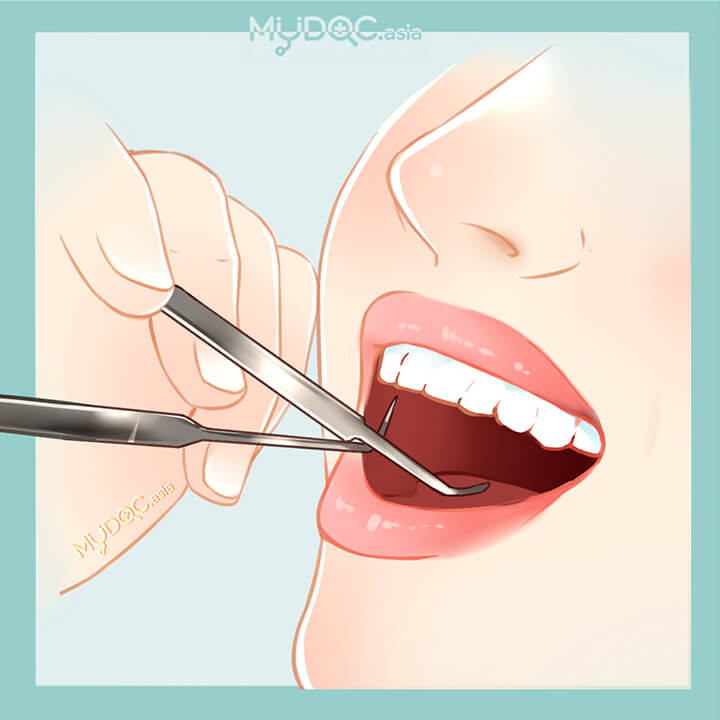• 牙科疾病

拔牙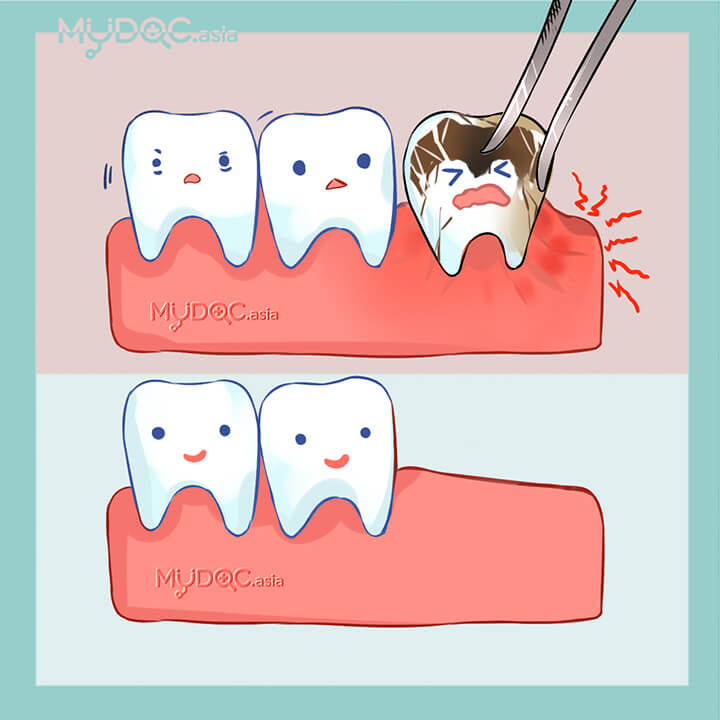• 蛀牙
• 牙齿松动

拔智慧牙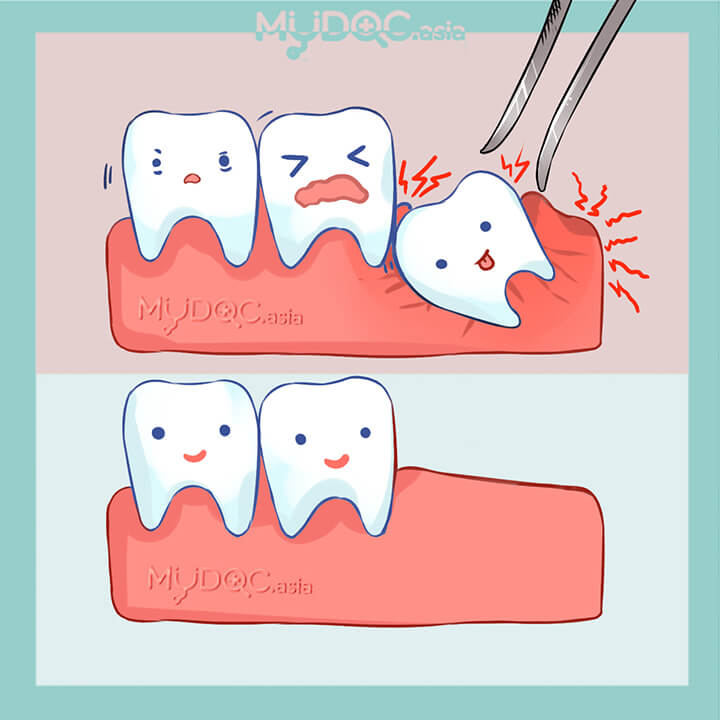• 智慧牙痛

断牙髓治疗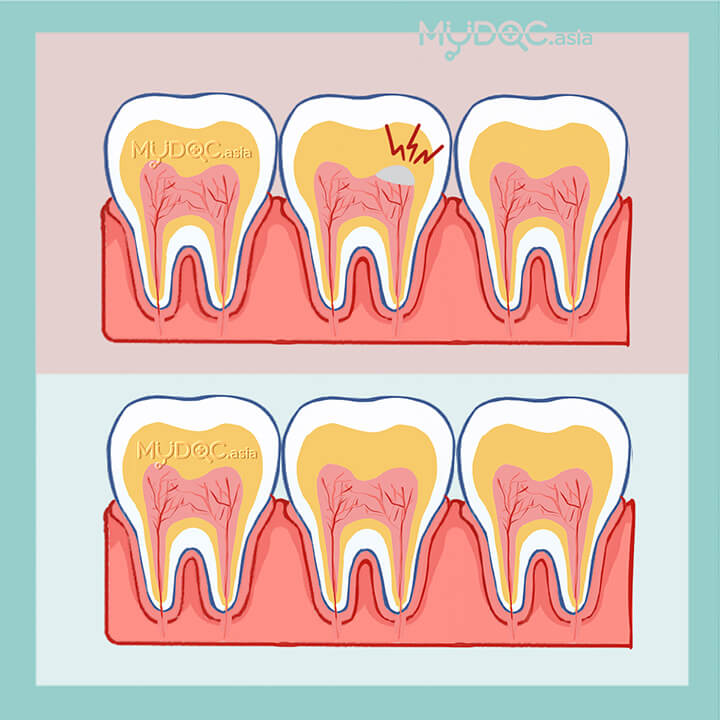• 牙髓病
• 蛀牙
• 牙齿损伤
• 牙齿感染
• 牙齿受伤
• 牙齿外伤

护牙套• 磨牙
• 睡眠呼吸暂停
• 颞颌关节症（TMJ）
• 体育相关的牙齿损伤
• 牙齿不均匀
• 深覆牙合
• 反颌咬合
• 咀嚼问题
• 弯曲的牙齿

护齿套• 磨牙

牙龈病治疗• 牙龈炎
• 牙龈感染
• 牙龈炎
• 牙龈出血
• 牙龈疼痛
• 牙龈问题

牙根切除手术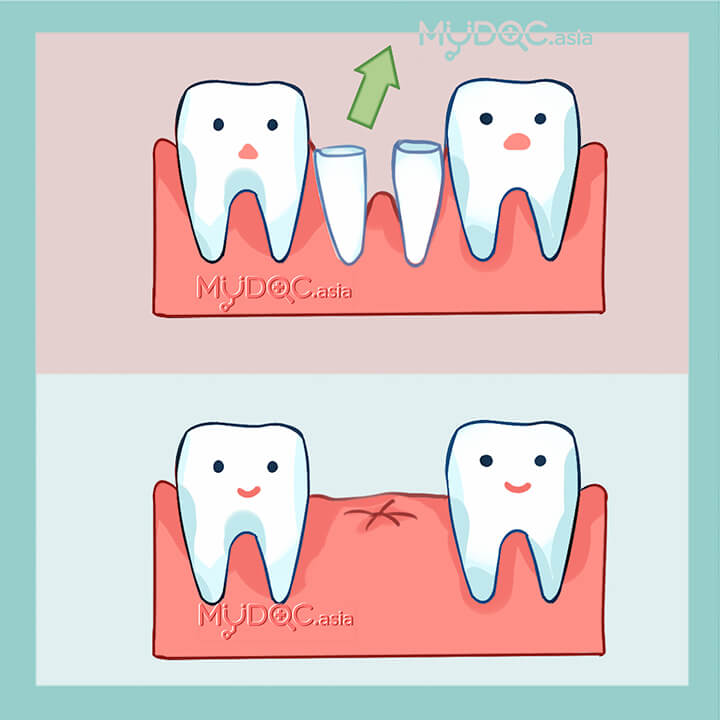• 蛀牙
• 牙髓病
• 牙根炎
• 牙根感染

拔牙（外科）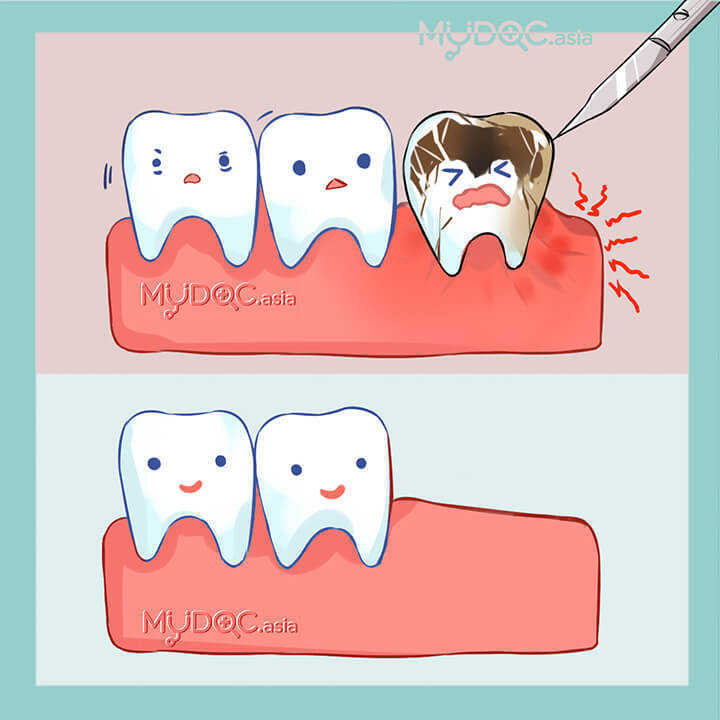• 蛀牙
• 牙齿松动

牙科X光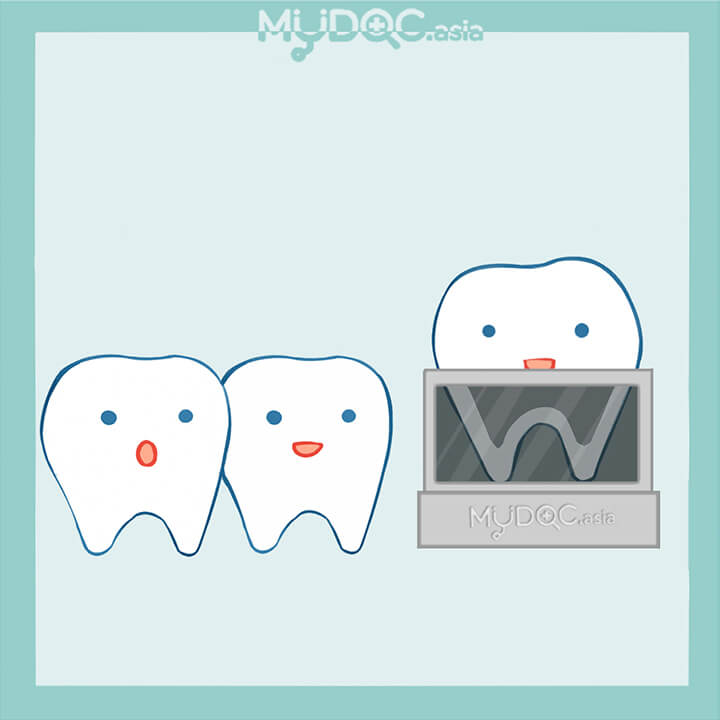• 牙科疾病
• 颚骨不均匀
• 颌关节损伤
• 牙齿外伤
• 牙齿受伤
• 牙关紧闭症

牙科全景X光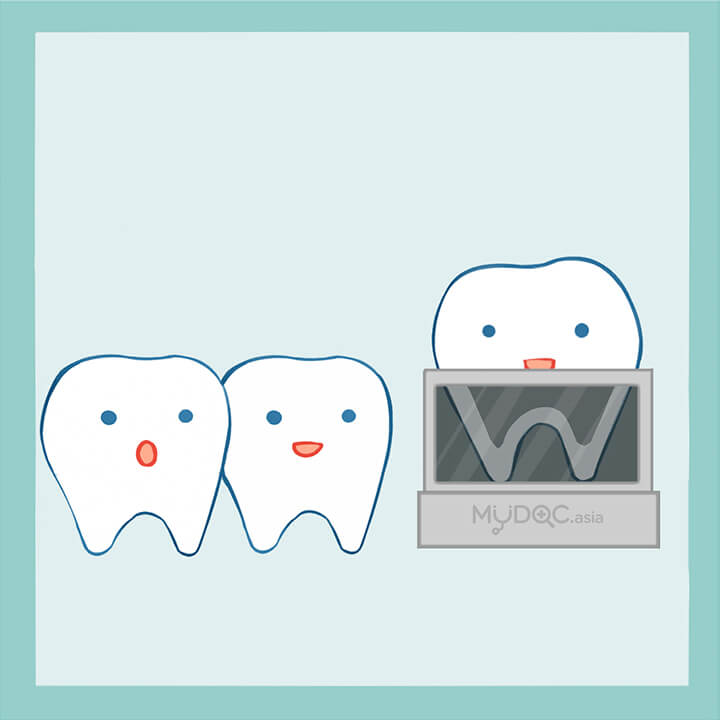• 牙科疾病
• 颚骨不均匀
• 颌关节损伤
• 牙齿外伤
• 牙齿受伤
• 牙关紧闭症

种植牙• 牙齿碎裂
• 缺牙
• 牙齿脱落
• 更换牙齿

迷你植牙• 牙齿脱落
• 缺牙
• 更换牙齿

钛合金种植牙• 牙齿脱落
• 缺牙
• 更换牙齿

All on4 全口植牙• 牙齿脱落
• 缺牙
• 更换牙齿

All on 6 全口植牙• 牙齿脱落
• 缺牙
• 更换牙齿

种牙式覆盖义齿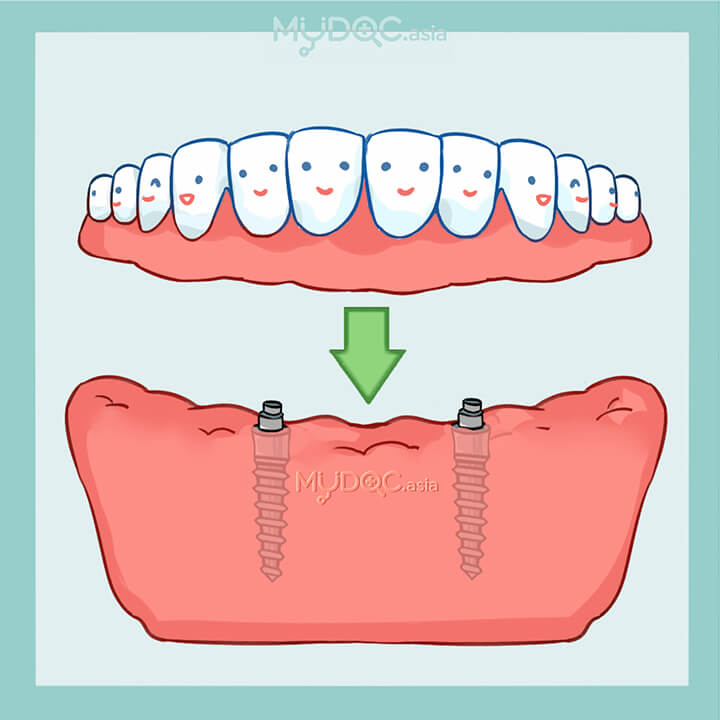• 牙齿脱落
• 缺牙
• 更换牙齿

拔智慧牙• 智慧牙痛

评论 我们的评论政策

5.0
★★★★★

评级分布
5 星
52
4 星
2
3 星
0
2 星
0
1 星
0
★★★★★ • Erufu Care 社区

★★★★★ • Erufu Care 社区

RM 1,070
★★★★☆ • Erufu Care 社区

RM 850

Andy Ooi博士然而李...我关心牙科康诺“很棒的服务和友好的员工！在设施方面超出了我的期望。安迪博士是一位真正的专业人士！ “我可能是世界上最严重的牙科病人之一。我很紧张，我唠叨而且我在磨。我有一个非常棒的医生“我的智慧牙齿已经插入了这里。经验丰富，无肿胀。熟练的医生和良好的火车工作人员 • 由谷歌翻译自英语原文

iCare Dental Connaught的丰富经验。我对团队提供的优质服务和友好态度感到满意。特别感谢Andy博士的专业精神。极力推荐。 • 由谷歌翻译自英语原文

Tq非常感谢Beh博士，Andy博士和团队......！你们真的很专业......！肯定会推荐给我的朋友和家人......！ • 由谷歌翻译自英语原文

★★★★☆ • dentaldepartures.com